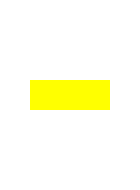# Web Puzzles

Showing posts with label carpet layer. Show all posts

## Carpet Layer

Walter Wall is a carpet layer. He and his two apprentices are asked by a nightclub owner to give a quote on laying carpet.

The owner indicates an oblong dance floor (figure on the right) and tells them that he wants a square of carpet adjacent each of the sides and running its entire length, making four squares in all (figure below left).

Walter asks both apprentices how many measurements must be made to calculate the total area of carpet needed in order to give a quote.Sam, the slower of the two, replies that eight measurements are needed: two sides of each square.Walter reprimands him, reminding him that these are squares and therefore have all sides the same length, and that they are in identical pairs, "So we only need to take two measurements - one side of one of the large squares and one side of one of the smaller squares".

Brian, the bright apprentice, points out that they can give the quote after taking only one measurement.

How can the total area (that is, the sum of the areas of the four red squares) be calculated by taking just one measurement?

### Carpet Layer Puzzle Solution

The only measurement that needs to be taken is the distance between opposite angles of the rectangular dance floor (figure on the left). That distance then will be squared (figure on the right) and doubled to get the sum of the areas of the 4 squares.